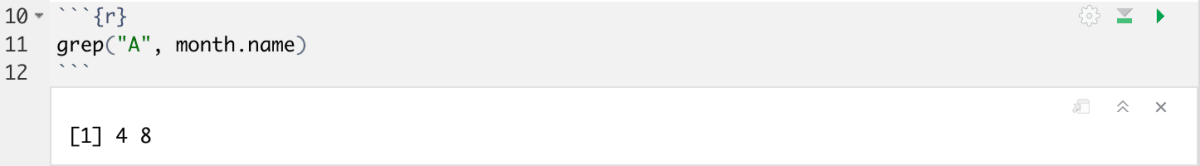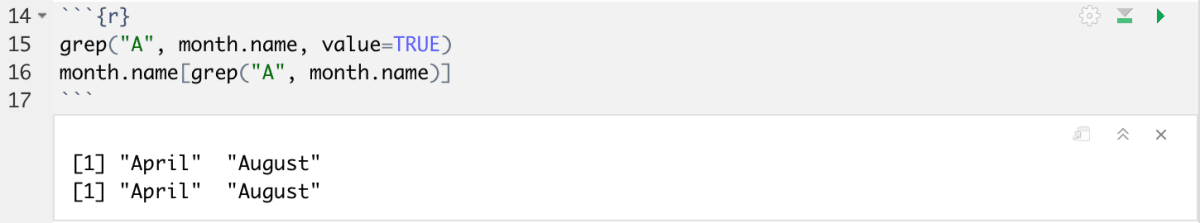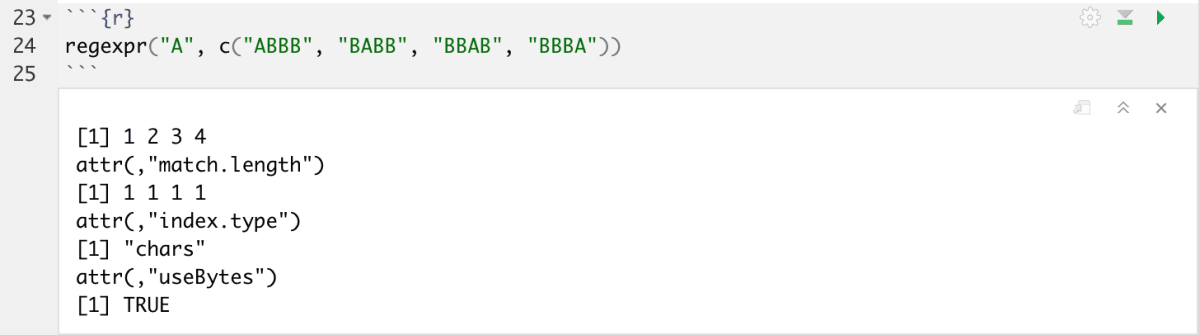Skip to content

# Regex

Regular expressions are used to search for a particular statement within an input string. When working with a large dataset, it may be useful to perform large scale searches, find patterns and perform substitutions. You can use the functions below to do so.

To understand the general methods for using regex in R, you can open the help page by running this command `?regexp`

Example: we will be using a vector, `month.name` and performing some searches on the values. `month.name` is a small dataset that contains the names of each month.

The `grep` function can be used to find and return the index of a match in a string.  We are searching for the capital `A` and returning the matches.

## Grep

`grep("A", month.name)`The `grep` function described above would return the indices of a positive match, in this case `>4 8`

To return the actual name, you can use the following statements:

`value=TRUE` prints the value itself

```grep("A", month.name, value=TRUE)
month.name[grep("A", month.name)]```

Both of these should return the names of the months that start with ‘A’Let’s look at a few more examples of regex commands. The syntax of these commands is similar to the grep function.

## Grepl

`grepl` returns a boolean, TRUE or FALSE depending on match.

`grepl("A", month.name)`## Regexpr

The `regexpr` function returns an integer vector of the position of the match. To understand the scope of this function, I’ve changed the input string used.

`regexpr("A", c("ABBB", "BABB", "BBAB", "BBBA"))`We can see that it returns a few statements. The first one is the position of the match(match.length)```  1 2 3 4 attr(,"match.length") ```

The second is an index of whether it is present or not. 1 being true and -1 being false.

``` 1 1 1 1 attr(,"index.type")```

The last line of the output, ‘chars’ is telling you the type of input string```  "chars" attr(,"useBytes")  TRUE```

## Gsub

The `gsub` function is used to substitute a string or part of a string with a replacement. The syntax is as follows:
`gsub('search', 'replacement', input)`

Example: I am substituting a part of some words to modify their spellings.
Creating a string called x:

```x <- c("Colour", "Flavour","Humour","Labour","Neighbour")
x```

Substituting `our` with `or`

`gsub('(our)', 'or', x, perl = TRUE)`

The result is as follows: